﻿ Getting Started Tutorials - MechDesigner > Tutorial 5: Kinematic Function Blocks for Motion > Step 5.6: Gearing, Amplitude and Frequency

# Step 5.6: Gearing, Amplitude and Frequency

## Amplitude and Frequency

This topic is: How to use the Gearing-Ratio parameter in the Gearing FB to edit the Amplitude and Frequency of motion-values.

We will use the Gearing-Ratio parameter. The motion-values in this tutorial are given to a rotating part we call a Rocker or Crank.

Note:  We use the term Crank when the kinematic-element Rocker rotates continuously.

#### Crank speed: Rotate a Crank at a speed that is different to the MMA

This is the most basic application. A crank rotates continuously, and we want it to rotate at a different speed or direction.

 We use a Gearing FB to change the angular velocity of a Crank. Connect a Linear Motion FB to a Gearing FB to a Motion-Dimension FB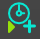---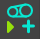---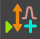There are two 'Cranks' in the image. The right-crank has a Gearing FB. Left Crank: The Linear-Motion FB increases linearly from 0 to 360, and repeats. Thus, the Crank rotates Counter-Clockwise at a constant speed, equal to the MMA. Note: Counter-Clockwise is the standard positive direction. Right Crank: Edit the Gearing FB to edit the Gearing Ratio parameter. Gearing Ratio: = -1 : The output = 0 to –360. The Crank rotates Clockwise each machine-cycle.   Gearing Ratio: = 3 - the output is now 0 to 1080. The crank rotates 3x for each machine-cycle.

#### Crank on Crank++We can add a crank to a crank.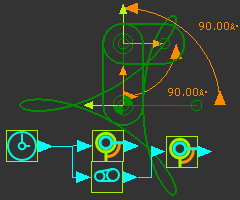Shown to the left is an Epicycloid Curve. To do this: 1.Join a Part to the Base-Part with a Pin-Joint. 2.Join a new Part to the end of the first Part with Pin-Joints 3.Use Motion-Dimension FBs fix the start angle of the two Parts. 4.Drive the Part joined to the Base-Part with a Linear-Motion FB – it is a Crank. 5.Drive the second Part, with a Gearing FB, and edit its Gearing Ratio to -3. 6.Use a Trace-Point to trace the path of the Point at the end of the second Part Note: You can easily replicate this motion with a Gear-Pair.

#### Gearing FB : Motion Amplitude AND Frequency

 There are three ways to use a Gearing FB. We can connect Gearing FBs to the input or the the output of a Motion FB. A Gearing FB joined to the: •input a Motion FB will change the frequency of the Motion •output a Motion FB will change the amplitude of the Motion •input and output will change the magnitude and frequency of a motion. Also, we can use a Gearing FB to change the direction of a motion. AMPLITUDE:Here, we connect a Motion FB to the input of the Gearing FB. For example: •Linear-Motion FB range = 0 to 360º •Motion FB range = 0 to 90mm •Gearing Ratio = '3'... The Gearing FB changes the AMPLITUDE of the Slider 0 to 270mm FREQUENCY:Here, we connect the Gearing FB to the input of the Motion FB. For example: •Linear-Motion FB range = 0 – 360º •Gearing Ratio = '3', •Input to Motion FB: range 0 – 1080º •Output from Motion FB range = 0 – 90mm 3 times in one machine-cycle. The Gearing FB increases the FREQUENCY of the Sliders motion by 3x Note - if the Gearing FB is '–ve', the input to the Motion FB is 0 to -360 thus the Motion is 'played' in reverse. AMPLITUDE AND FREQUENCY:To change the amplitude and frequency of a motion, connect a Gearing FB before and after a Motion FB.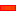VIDEOS :